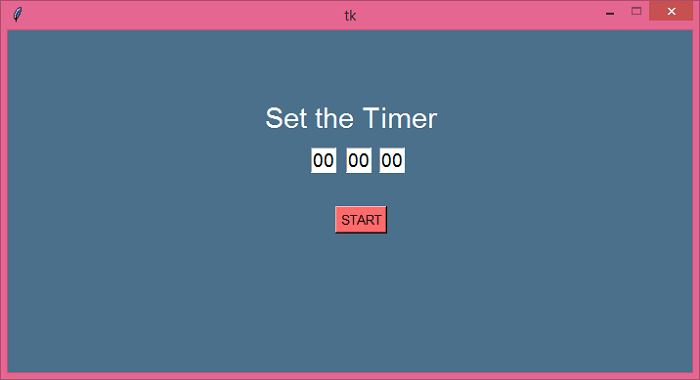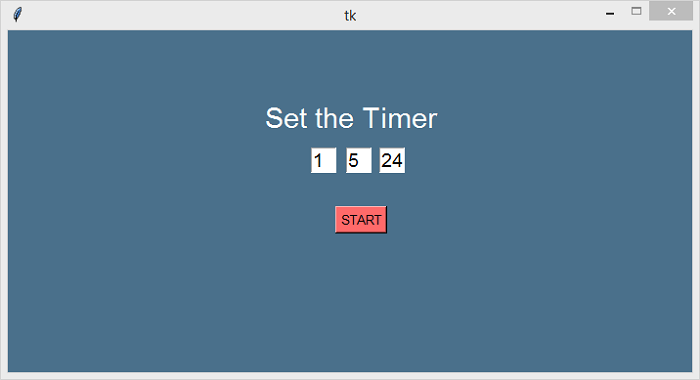# Making a countdown timer with Python and Tkinter

Tkinter is a standard Python library for creating GUI-based desktop applications. It offers a variety of functions, modules, and methods that can be used to implement the functionality of an application.

In this example, we will create a countdown Time using Python standard libraries such as Tkinter and time module. The basic functionality of our application would be to run the timer for a given period of time. It will have the following components,

• An Entry widget to set the timer each for HH/MM/SS.

• A Button to execute the function countdowntimer().

• A function countdowntimer() will convert the input string into an integer value relative to the HH, MM, and SS.

• Using update() method, we will update the window with respect to the given function and widgets.

## Example

# Import the required library
from tkinter import *
import time

# Create an instance of tkinter frame
win = Tk()

# Set the size of the window
win.geometry('700x350')

# Make the window fixed to its size
win.resizable(False, False)

# Configure the background
win.config(bg='skyblue4')

# Create Entry Widgets for HH MM SS
sec = StringVar()
Entry(win, textvariable=sec, width=2,
font='Helvetica 14').place(x=380, y=120)
sec.set('00')

mins = StringVar()
Entry(win, textvariable=mins, width=2, font='Helvetica 14').place(x=346, y=120)
mins.set('00')

hrs = StringVar()
Entry(win, textvariable=hrs, width=2, font='Helvetica 14').place(x=310, y=120)
hrs.set('00')

# Define the function for the timer
def countdowntimer():
times = int(hrs.get()) * 3600 + int(mins.get()) * 60 + int(sec.get())
while times > -1:
minute, second = (times // 60, times % 60)
hour = 0
if minute > 60:
hour, minute = (minute // 60, minute % 60)
sec.set(second)
mins.set(minute)
hrs.set(hour)

# Update the time
win.update()
time.sleep(1)
if (times == 0):
sec.set('00')
mins.set('00')
hrs.set('00')
times -= 1

# Create a Label widget
Label(win, font=('Helvetica bold', 22), text='Set the Timer', bg='skyblue4', fg="white").place(x=260, y=70)

# Button widget to set the timer
Button(win, text='START', bd='2', bg='IndianRed1', font=('Helvetica bold', 10), command=countdowntimer).place(x=335, y=180)

win.mainloop()

## Output

It will display a countdown timer in the window.If we set the timer by changing the value in the Entry boxes and click the "start" button, it will quickly start the timer for the given period of time.